# Closed Shapes

Go back to  'Solid Shapes'

## Triangles

### What is a triangle?

A triangle is a closed figure with 3 sides and 3 vertices.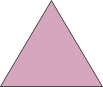## Classification of triangles by sides

### Equilateral triangle

If all the three sides of a triangle are equal, it is called an equilateral triangle.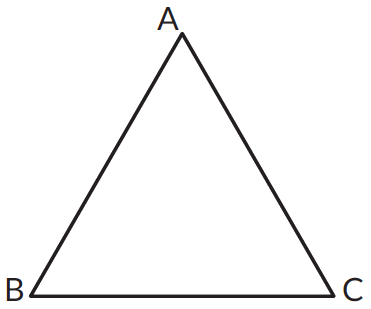All three sides are equal in length. $$AB = BC = AC$$

### Isosceles triangle

If any two sides of a triangle are equal, it is called an isosceles triangle.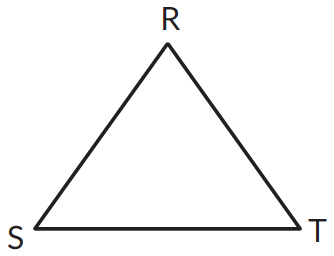Two sides of this triangle are equal. $$RS = RT$$

### Scalene triangle

If a triangle has three unequal sides, it is called a scalene triangle.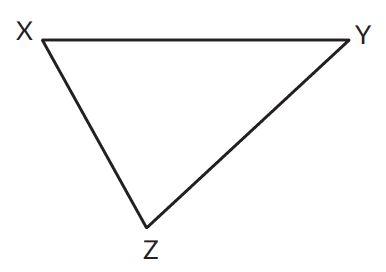In this triangle all three sides are of unequal lengths. $$XZ ≠ XZ ≠ YZ$$

## Classification of triangles by angles

### Right angled triangle

If any angle of a triangle is $$90^\circ,$$ the triangle is called a right-angled triangle or a right triangle.$$\angle ABC = \,90^\circ.$$ Therefore $$\triangle ABC$$ is a right angled triangle.

### Obtuse angled triangle

If any angle of a triangle is obtuse, then the triangle is called an obtuse-angled triangle or an obtuse triangle.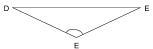$$\angle DEF$$ is an obtuse angle. Therefore $$\triangle DEF$$ is an obtuse-angled triangle.

### Acute angled triangle

If every angle of a triangle is acute, then the triangle is called an acute-angled triangle or an acute triangle.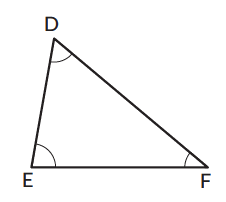All the angles, $$\angle DEF,\;\angle EFD,$$ and $$\angle EDF$$ are all acute angles. Therefore, the triangle is an acute-angled triangle.

## Procedure - Angle sum property of a triangle

The sum of the angles of a triangle is $$180^\circ.$$ This property is called the angle sum property of a triangle. All the interior angles sum up to $$180^\circ.$$

Let’s try and understand this with the help of an example.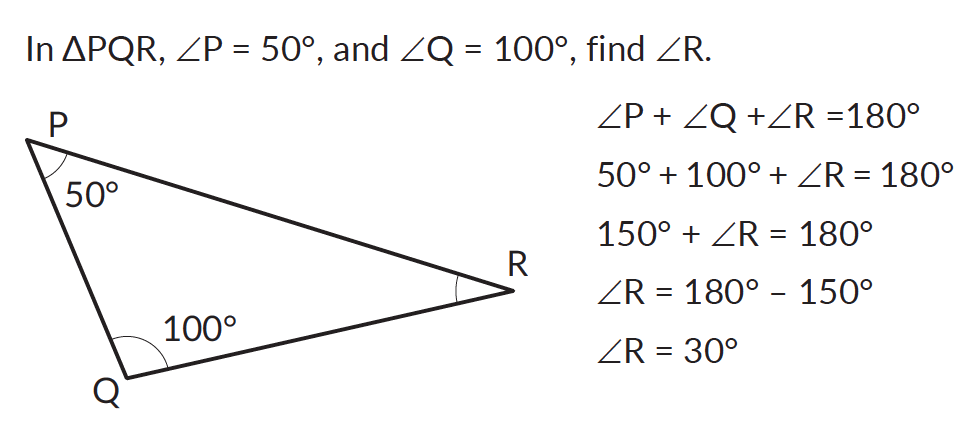## Circles

### Circle

In the figure, $$P$$ is the centre of the circle. The centre of a circle is a point within the circle from which every point on the circle is at the same distance.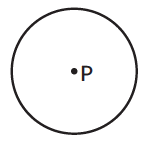The radius of a circle is the distance from the centre of the circle to any point on the circle. $$PQ$$ is the radius of the circle. Infinite number of radii can be drawn for any circle.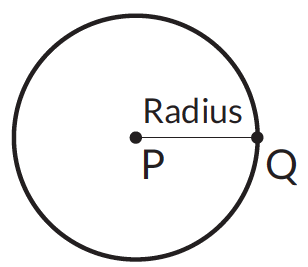### Circumference

The circumference of a circle is its boundary.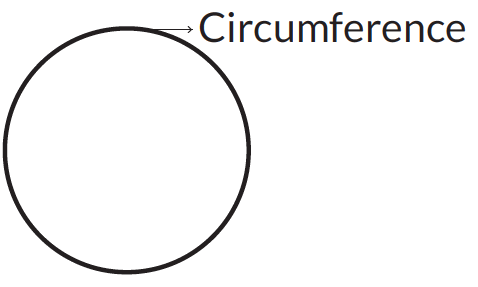### Arc

An arc is a portion of the circumference of the circle. The figure shows both the minor arc as well as the major arc of the circle.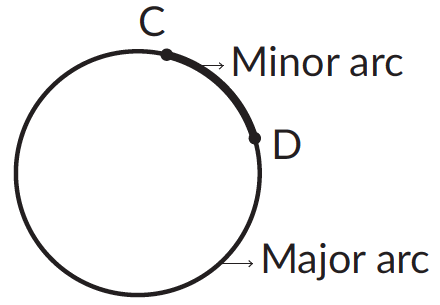### Chord

A chord of a circle is a line segment whose end-points lie on the circumference.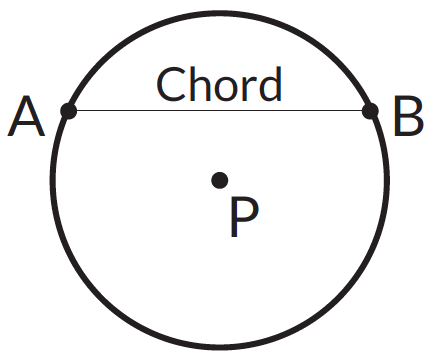### Diameter

The diameter of a circle is a chord that passes through the centre of the circle. $$AB$$ is the diameter of the circle.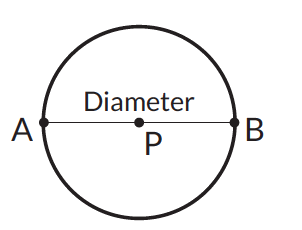### Segments of the circle

A chord of a circle divides the circle into two parts, which are called segments of the circle.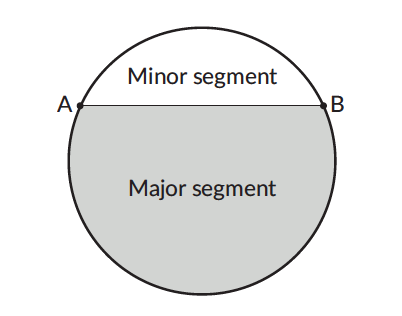A sector of a circle is the region enclosed by two radii and an arc as shown.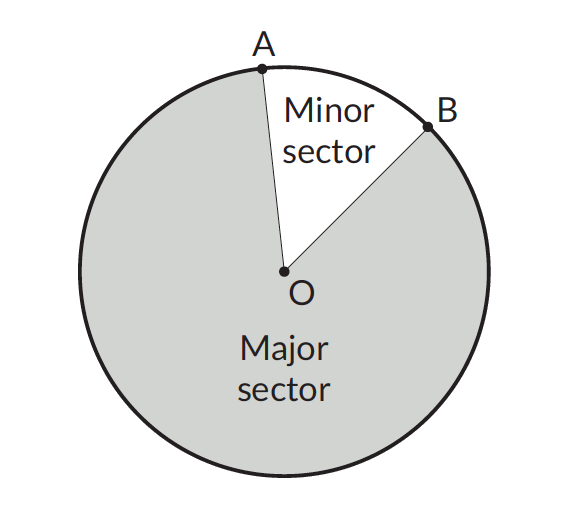## Construction of a circle?

1. Setting a compass to a given radius, e.g., 4.2 cm.

Step 1 - set the needle of the compass on the zero mark of the ruler.
Step 2 - extend the other arm of the compass so that the pencil's tip lands on the required mark, i.e., 4.2 cm.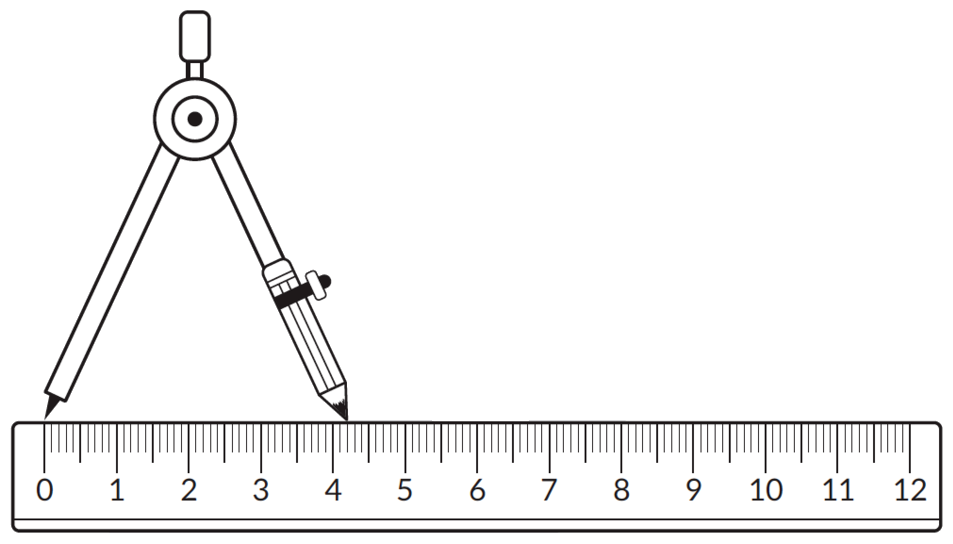2. Measuring the length of a line segment using a compass and a ruler.

Step 1 - Set the needle on one end point (say $$A$$) of the line segment.
Step 2 - Extend the other arm of the compass so that the pencil's tip lands on the other end point $$(B).$$Step 3 - Without changing the radius of the compass, place both its ends on the ruler with the needle on the zero mark.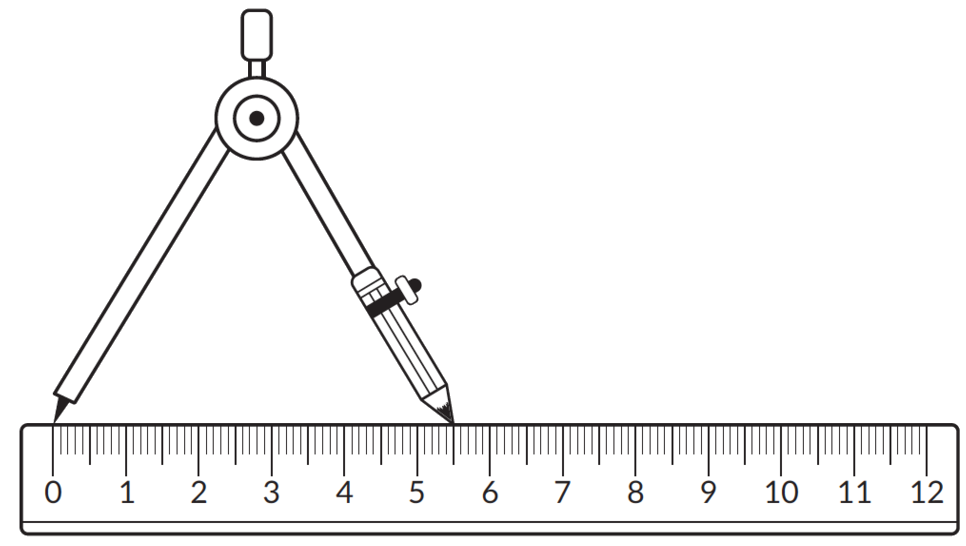-  The mark on which the pencil's tip lands is the length of the line segment.

3. Drawing a circle of a given radius with a given point as the centre.
For example, let's draw a circle of radius 2.5 cm using $$A$$ as the centre

Step 1 - Use the ruler to set the compass to the required radius, i.e., 2.5 cm
Step 2 - Keeping the needle at $$A$$ and the pencil’s tip on the paper, turn the compass about $$A$$ to draw a closed curve.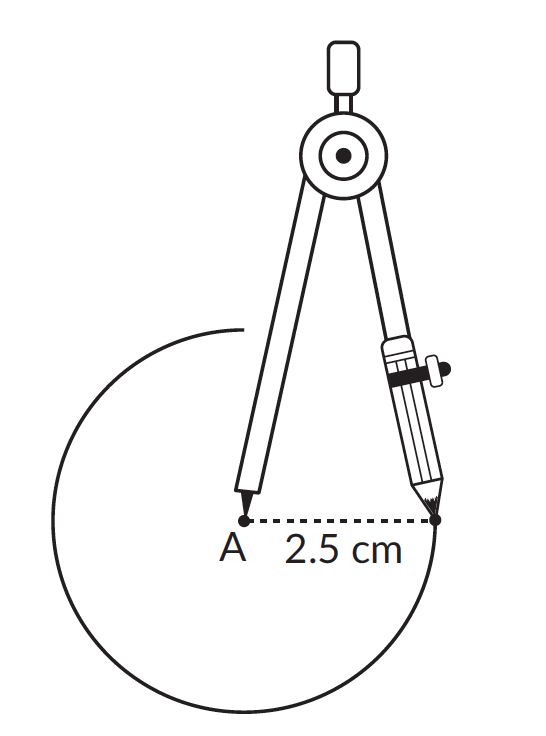-  The circle formed has its centre at A and has a radius of 2.5 cm

## Formulas

Given below are the formulas for different features of a circle. The value of $$\pi$$ can be approximated to \begin{align}22\over 7 \end{align} or $$3.14$$.

 Circumference / Perimeter $$2\pi r$$ While calculating the circumference the formula can also be written as $$\pi d.$$ ‘$$d$$’ is the diameter and the formula for it is $$2r$$ Diameter $$2r$$ Diameter is twice the radius of the circle. Area $$\pi r^2$$ It is $$\pi$$ times the radius squared. Area when the diameter is given\begin{align} A={\pi \over 4} \times D^2. \end{align} Area when the circumference is given \begin{align}= A={C^2 \over 4 \pi}. \end{align}

A quadrilateral is a polygon with 4 sides and 4 vertices.

### SquareProperties of a square

A square has

• four equal sides - $$\text { Side } AB = \text { Side } BC = \text { Side } CD = \text { Side } AD$$
• four right angles - $$\angle A = \angle B = \angle C = \angle D =\, 90^\circ$$
• two pairs of parallel sides - $$AB ∥ DC \qquad AD ∥ BC$$

### RectangleProperties of a rectangle

A rectangle has

• two pairs of parallel sides. $$AB ∥ DC \qquad AD ∥ BC$$
• four right angles. $$\angle A = \angle B = \angle C = \angle D =\, 90^\circ$$
• opposite sides of equal length. $$AD = BC$$ & $$AB = DC$$

### ParallelogramProperties of a parallelogram

• two pairs of parallel sides $$PQ ∥ RT \qquad RT ∥ QT$$
• opposite sides are of equal length. $$\text{ Side } PQ = \text{ Side } RT$$ and $$\text{ Side } PR = \text{ Side } QT$$

### Trapezium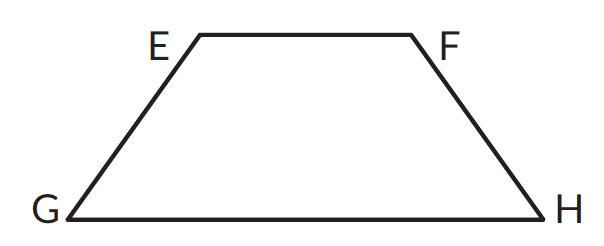Properties of a trapezium

• One pair of parallel sides. $$EH ∥ GH$$

### Rhombus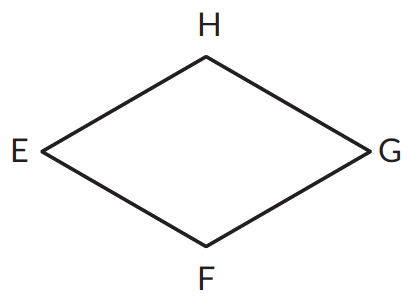A rhombus has

• two pairs of parallel sides. $$EH ∥ FG \qquad EF ∥ HG$$
• four equal sides. $$\text{ Side } EH = \text{ Side } HG = \text{ Side } GF = \text{ Side } FE$$
• Opposite angles are equal. $$\angle H = \angle F = \angle E = \angle G$$

## Angle sum property of quadrilaterals

The sum of the angles of a quadrilateral is always $$360^\circ.$$ This property is referred to as the angle sum property of a quadrilateral.In the figure given above the sum of angles $$A,\;B,\;C$$ & $$D$$ is $$360^\circ.$$ This property comes handy when calculating unknown measure of angles in a quadrilateral.

## Tips and Tricks

• The value of $$\pi$$ can be approximated as \begin{align}22\over 7\end{align} when the radius of the circle is divisible by 7. Otherwise it can be approximated to 3.14. This can be used to calculate the area or the circumference.
• While calculating the area of a circle it is helpful to write the radius twice instead of squaring it. Squaring would make the calculations tedious. This holds true for radius with larger values. For example, if the radius of a circle is 21 cm it would be easier to calculate \begin{align}{22\over 7} \times 21 \times 21\end{align} than calculating \begin{align}{22\over 7} \times 441.\end{align}
• Tip: While naming a quadrilateral you can name it in anti-clockwise direction. For example, in the figure given below the quadrilateral can be named as $$\square ABCD$$ and $$\square ACBD.$$• While calculating the measures of an unknown angles in a quadrilateral dividing the quadrilateral into two triangles would help.

### Common mistakes or misconceptions

Misconception 1: Any given chord is a diameter

A diameter is a chord, as it is a line segment whose end-points lie on the circumference. However not all chords are diameters, as a chord may or may not pass through the centre of the circle.

Misconception 2: Any four sided figure is a quadrilateral. For example, the figure given below is a quadrilateral.Though the above give has 4 sides and is a closed figure it is not a quadrilateral. Quadrilaterals are closed figures made of non-intersecting line segments.

Misconception 3: All rectangles are squares

Many rectangles do not have 4 equal sides and 4 equal angles. However all squares are rectangles as they all have 4 right angles, their opposite sides are parallel to each other and opposite sides are equal to each other.

Misconception 4: All rhombuses are squares

Many rhombuses do not have four equal sides and four equal angles. However all squares are rhombuses as it has 4 equal sides, its opposite sides are parallel to each other.

## Depict the following

1.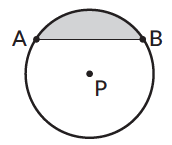The shaded portion is a ________________.

2.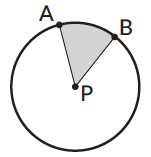The shaded portion is a ________________.

3.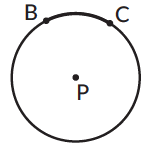BC is ______________________________.

More Important Topics
Numbers
Algebra
Geometry
Measurement
Money
Data
Trigonometry
Calculus
More Important Topics
Numbers
Algebra
Geometry
Measurement
Money
Data
Trigonometry
Calculus
0